Updating search results...

# 6 Results

View
Selected filters:
• Conceptual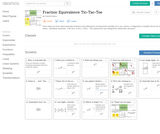Rating
0.0 stars

Show what you know about equivalent fractions and ordering by choosing three activities (in a row, column, or diagonal) to complete the tic-tac-toe board. Standards assessed: 3.NF.3a-d, 4.NF.1, 4.NF.2. This assessment was designed for adult basic education.

Subject:
Mathematics
Numbers and Operations
Material Type:
Assessment
Interactive
Author:
Connie Rivera
11/10/2017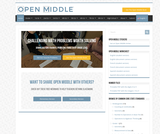Conditional Remix & Share Permitted
CC BY-NC-SA
Rating
0.0 stars

Challenging math problems worth solving.

The name “Open Middle” might sound like a strange name for a website about math problems. However, it references a very specific type of problem we try to encourage here. Most of the problems on this site have:
* a “closed beginning” meaning that they all start with the same initial problem.
* a “closed end” meaning that they all end with the same answer.
* an “open middle” meaning that there are multiple ways to approach and ultimately solve the problem.

Open middle problems generally require a higher Depth of Knowledge than most problems that assess procedural and conceptual understanding. They support the Common Core State Standards and provide students with opportunities for discussing their thinking.

Subject:
Mathematics
Material Type:
Activity/Lab
Lesson
Author:
Nanette Johnson
Open Middle
Robert Kaplinsky
03/17/2020
Remix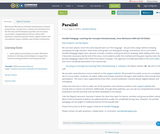Only Sharing Permitted
CC BY-ND
Rating
0.0 stars

Newtonian Mechanics whereby fundamental concepts (momentum, energy, force, motion) are introduced on the first day and developed in parallel. Access entire curriculum: comprehensive lecture videos with questions, textbook (calculus based, algebra based, and conceptual), exams, syllabus, past student evaluations.

Subject:
Physics
Material Type:
Activity/Lab
Assessment
Full Course
Homework/Assignment
Interactive
Lesson Plan
Teaching/Learning Strategy
Textbook
Author:
Houda El Khoury
08/08/2019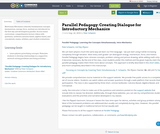Conditional Remix & Share Permitted
CC BY-SA
Rating
0.0 stars

Newtonian Mechanics whereby fundamental concepts (momentum, energy, force, motion) are introduced on the first day and developed in parallel. Access entire curriculum: comprehensive lecture videos with questions, textbook (calculus based, algebra based, and conceptual), exams, syllabus, past student evaluations.

Subject:
Physics
Material Type:
Activity/Lab
Assessment
Full Course
Homework/Assignment
Interactive
Lesson Plan
Teaching/Learning Strategy
Textbook
Author:
Peter Schwartz
08/24/2018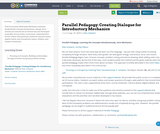Conditional Remix & Share Permitted
CC BY-SA
Rating
0.0 stars

Full Curriculum, Newtonian Mechanics whereby fundamental concepts (momentum, energy, force, motion) are introduced on the first day and developed in parallel. Access entire curriculum: comprehensive lecture videos with questions, textbook (calculus based, algebra based, and conceptual), exams, syllabus, past student evaluations.

Subject:
Physics
Material Type:
Activity/Lab
Assessment
Full Course
Homework/Assignment
Interactive
Lecture
Teaching/Learning Strategy
Textbook
Author:
Peter Schwartz
08/27/2018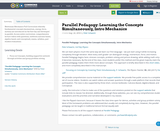Conditional Remix & Share Permitted
CC BY-SA
Rating
0.0 stars

Newtonian Mechanics Full Curriculum whereby fundamental concepts (momentum, energy, force, motion) are introduced on the first day and developed in parallel. Access entire curriculum: comprehensive lecture videos with questions, textbook (calculus based, algebra based, and conceptual), exams, syllabus, past student evaluations.

Subject:
Physics
Material Type:
Activity/Lab
Assessment
Full Course
Homework/Assignment
Lecture
Teaching/Learning Strategy
Textbook
Author:
Peter Schwartz